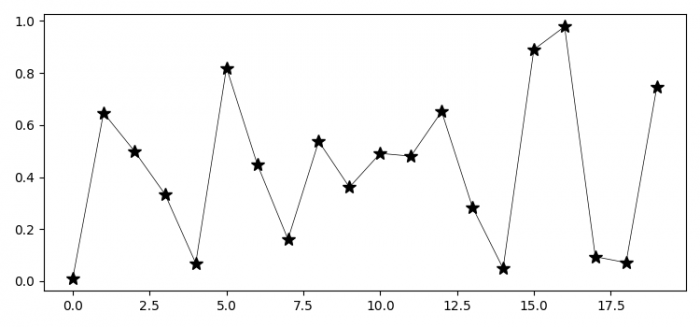# How to make markers on lines smaller in Matplotlib?

To make markers on lines smaller in Matplotlib, we can take the following steps −

• Set the figure size and adjust the padding between and around the subplots.
• Create random data points, x.
• Plot x data points using plot() method, with linewidth =0.5 and color="black".
• To display the figure, use show() method.

## Example

from matplotlib import pyplot as plt
import numpy as np

plt.rcParams["figure.figsize"] = [7.50, 3.50]
plt.rcParams["figure.autolayout"] = True

x = np.random.rand(20)
plt.plot(x, '*-', color='black', markersize=10, lw=0.5)

plt.show()

## Output